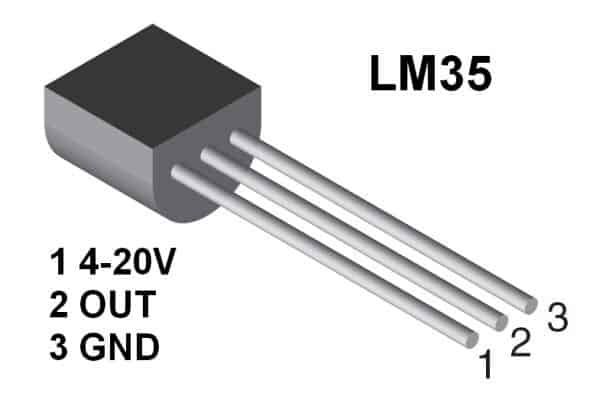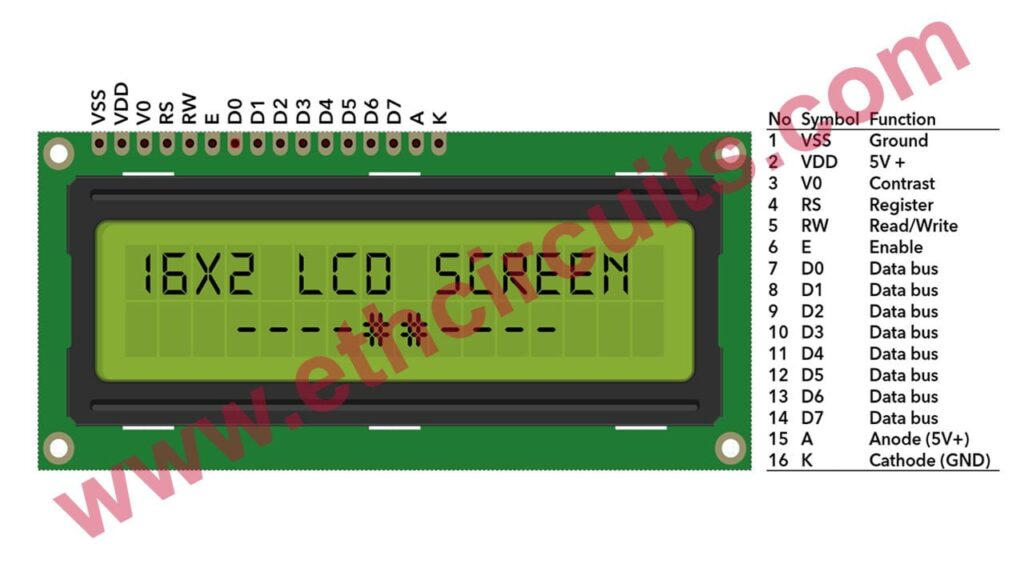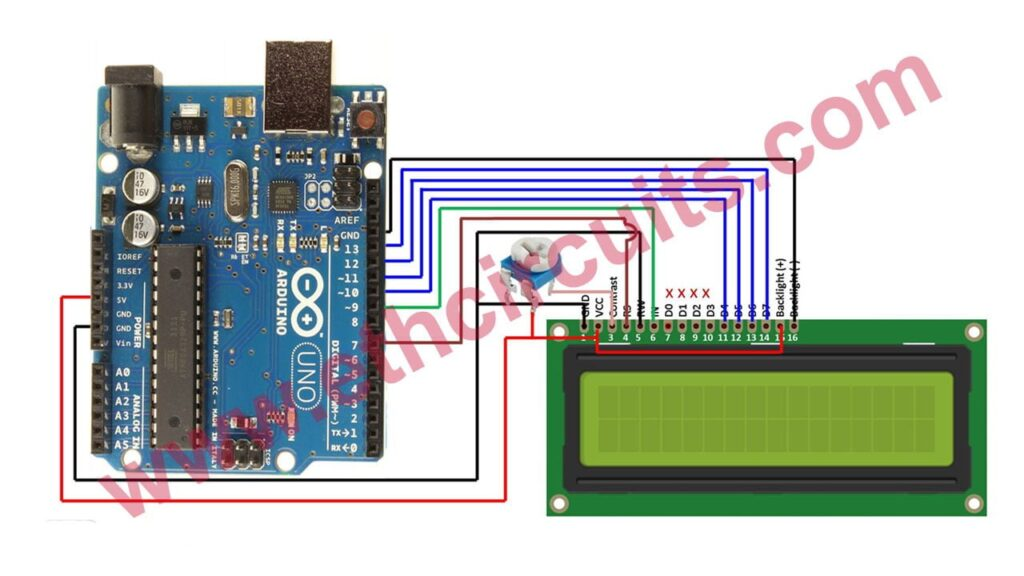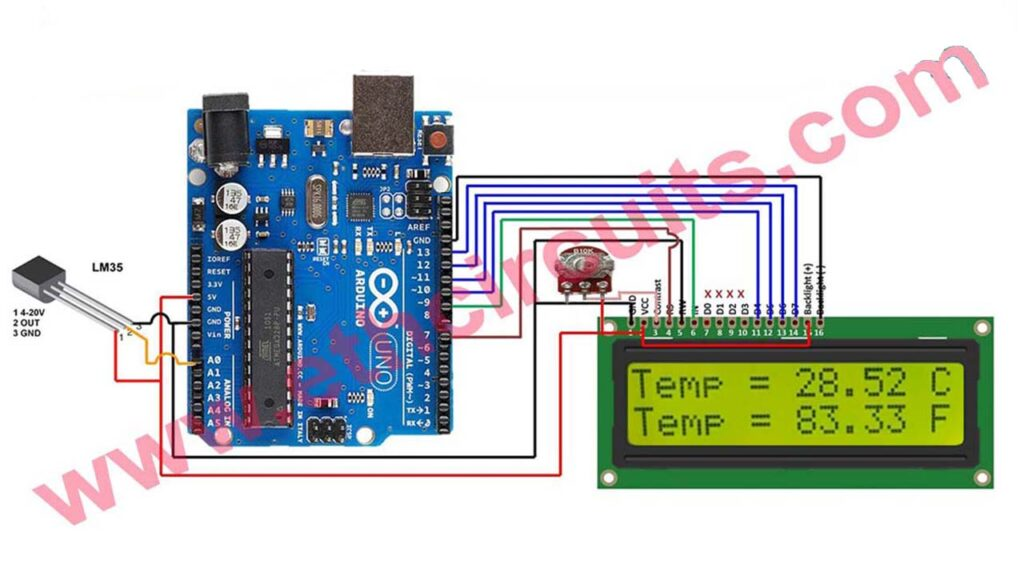# How To make Arduino Temperature Sensor

In this article, I will explain how to interface LM35 and Arduino along with its program. Once we successfully interface arduino and lm35, we will go on to build a temperature display using arduino and a 16×2 LCD module which constantly monitors temperature around the measurement field/range of LM35 and displays the same on LCD module. So lets get to building the Arduino Temperature Sensor.

We are using Arduino Uno as our board and LM35 can be connected to arduino as shown in circuit diagram.

## LM35 Introduction and Pinout

LM35 is an analog, linear temperature sensor whose output voltage varies linearly with change in temperature. LM35 is three terminal linear temperature sensor from National semiconductors.

It can measure temperature from-55 degree celsius to +150 degree celsius. The voltage output of the LM35 increases 10mV per degree Celsius rise in temperature. LM35 can be operated from a 5V supply and the stand by current is less than 60uA. The pin out of  LM35 is shown in the figure below.

LM35 is available in the market in 3 series variations – LM35A, LM35C and LM35D series. The main difference between these 3 versions of LM35 IC are in their range of temperature measurements.

The LM35D series is designed to measure from 0 degree Celsius to 100 degree Celsius, where as the LM35A series is designed to measure a wider range of -55 degree Celsius to 155 degree Celsius. The LM35C series is designed to measure from -40 degree Celsius to 110 degree Celsius## 16×2 LCD Module

We come across LCD displays everywhere around us. Computers, calculators, television sets, mobile phones, digital watches use some kind of display to display the time.

An LCD is an electronic display module that uses liquid crystal to produce a visible image. The 16×2 LCD display is a very basic module commonly used in DIYs and circuits. The 16×2 translates a display 16 characters per line in 2 such lines. In this LCD each character is displayed in a 5×7 pixel matrix.

## 16×2 LCD Module Pinout## Arduino Temperature Sensor Connection Diagram of LCD & Arduino

You can use any Arduino Digital pin with  LCD but you have to mention those pins in you coding, Follow this connection diagram shown in the figure below. I will use these pins in every of my Arduino LCD Project## Arduino Temperature Meter, LM35 Connection With Arduino UNO## Arduino Temperature Meter Code

Just Copy and Paste this code and upload to Arduino like my video

```#include <LiquidCrystal.h>

// initialize the library with the numbers of the interface pins
LiquidCrystal lcd(7, 9, 10, 11, 12,13);

//declare variables
float tempC;
float tempF;
int tempPin = 0;

void setup(){
// set up the LCD's number of columns and rows:
lcd.begin(16, 2);
lcd.print("Temp1=");
lcd.setCursor(0, 1);
lcd.print("Temp2=");
}

void loop(){
tempC = (5.0 * tempC * 100.0)/1024.0; //convert the analog data to temperature
tempF = ((tempC*9)/5) + 32; //convert celcius to farenheit

// print result to lcd display
lcd.setCursor(6, 0);
lcd.print(tempC,1);
lcd.print("'C");

lcd.setCursor(6, 1);
lcd.print(tempF,1);
lcd.print("'F");

// sleep...
delay(1000);
}```
error: Content is protected !!
Scroll to Top